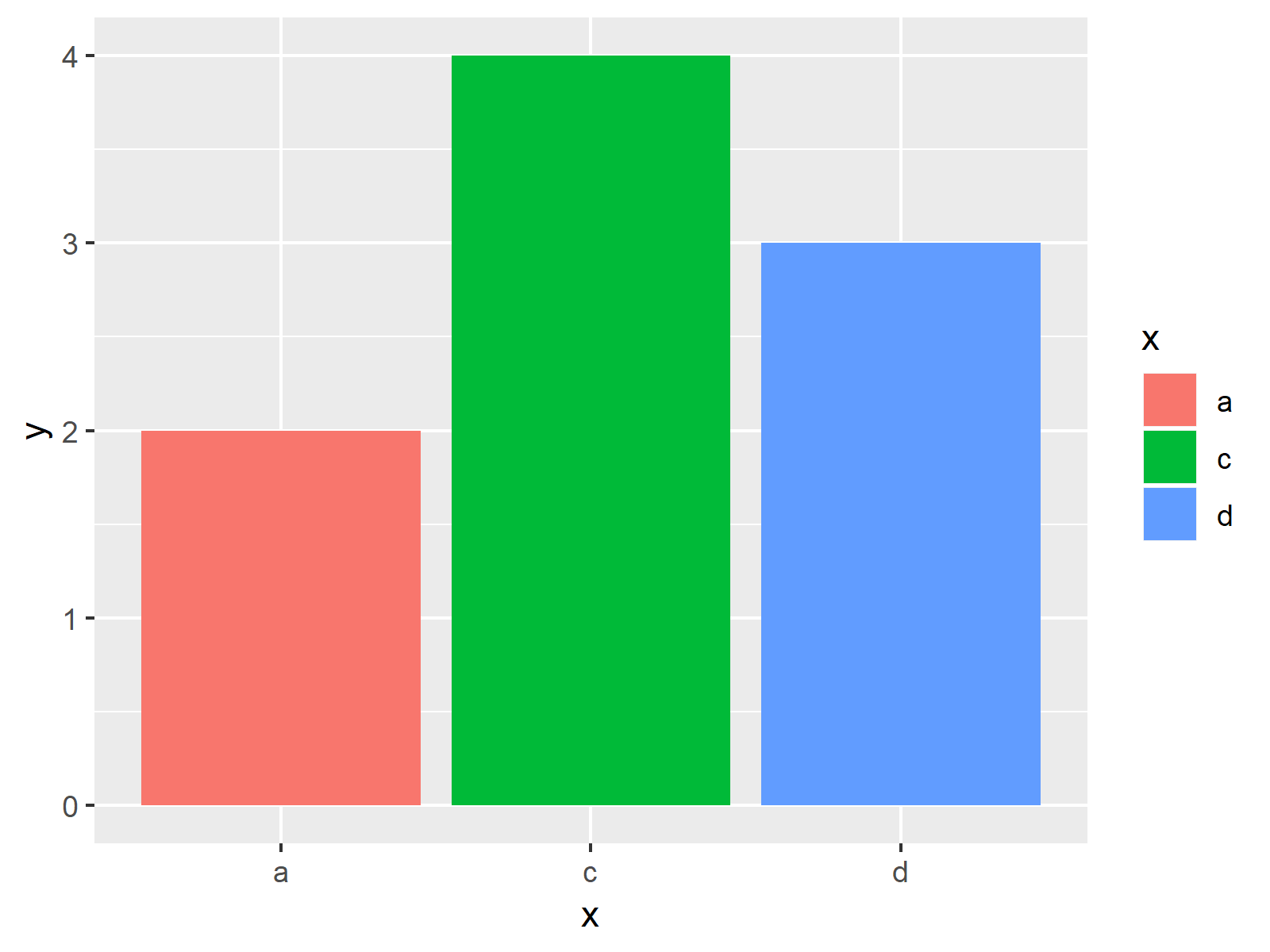# Keep Unused Factor Levels in ggplot2 Barplot in R (Example)

This tutorial shows how to draw a ggplot2 barplot containing empty factor levels in R programming.

The content of the tutorial is structured like this:

With that, let’s dive right in:

## Exemplifying Data, Add-On Packages & Basic Graph

As a first step, we’ll have to create some data that we can use in the example code later on:

```data <- data.frame(x = letters[1:5],          # Example data
y = c(2, 0, 4, 3, 0))
data <- data[data\$y > 0, ]
data                                          # Return data
#   x y
# 1 a 2
# 3 c 4
# 4 d 3```

The previous output of the RStudio console shows the structure of our example data – a data frame containing two columns. The first variable defines the groups of our barplot and the second variable the corresponding values.

If we want to plot the data with the ggplot2 package, we also need to install and load ggplot2:

```install.packages("ggplot2")                   # Install ggplot2 package

Now, we can draw the data as follows:

```ggp <- ggplot(data, aes(x, y, fill = x)) +    # Create barchart without 0-levels
geom_bar(stat = "identity")
ggp                                           # Draw barplot```Figure 1 illustrates the output of the previous R code: A barplot showing only factor levels with values larger than 1.

## Example: Keep Empty Factor Levels in Barplot

The R code below illustrates how to print a barchart that keeps factor levels with a value of 0. For this task, we have to use the scale_x_discrete function. Within the scale_x_discrete, we have to specify the argument drop to be equal to FALSE:

```ggp +                                         # Create barchart with empty factors
scale_x_discrete(drop = FALSE)```As shown in Figure 2, the previous R programming code created a barchart with empty factor levels.

## Video & Further Resources

Do you want to learn more about drawing barcharts with the ggplot2 package? Then you might watch the following video of my YouTube channel. I explain the R programming syntax of this article in the video:

Please accept YouTube cookies to play this video. By accepting you will be accessing content from YouTube, a service provided by an external third party.If you accept this notice, your choice will be saved and the page will refresh.

In addition, you might want to have a look at the related articles of my homepage.

In this article you learned how to create a barchart that shows empty factor levels in R programming. If you have additional comments and/or questions, let me know in the comments.

Subscribe to the Statistics Globe Newsletter